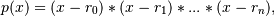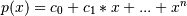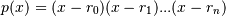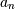# numpy.polynomial.polynomial.polyfromroots¶

`numpy.polynomial.polynomial.``polyfromroots`(roots)[source]

Generate a monic polynomial with given roots.

Return the coefficients of the polynomialwhere the r_n are the roots specified in roots. If a zero has multiplicity n, then it must appear in roots n times. For instance, if 2 is a root of multiplicity three and 3 is a root of multiplicity 2, then roots looks something like [2, 2, 2, 3, 3]. The roots can appear in any order.

If the returned coefficients are c, thenThe coefficient of the last term is 1 for monic polynomials in this form.

Parameters: roots : array_like Sequence containing the roots. out : ndarray 1-D array of the polynomial’s coefficients If all the roots are real, then out is also real, otherwise it is complex. (see Examples below).

`chebfromroots`, `legfromroots`, `lagfromroots`, `hermfromroots`, `hermefromroots`

Notes

The coefficients are determined by multiplying together linear factors of the form (x - r_i), i.e.where `n == len(roots) - 1`; note that this implies that 1 is always returned for.

Examples

```>>> from numpy.polynomial import polynomial as P
>>> P.polyfromroots((-1,0,1)) # x(x - 1)(x + 1) = x^3 - x
array([ 0., -1.,  0.,  1.])
>>> j = complex(0,1)
>>> P.polyfromroots((-j,j)) # complex returned, though values are real
array([ 1.+0.j,  0.+0.j,  1.+0.j])
```

#### Previous topic

numpy.polynomial.polynomial.polyroots

#### Next topic

numpy.polynomial.polynomial.polyvalfromroots